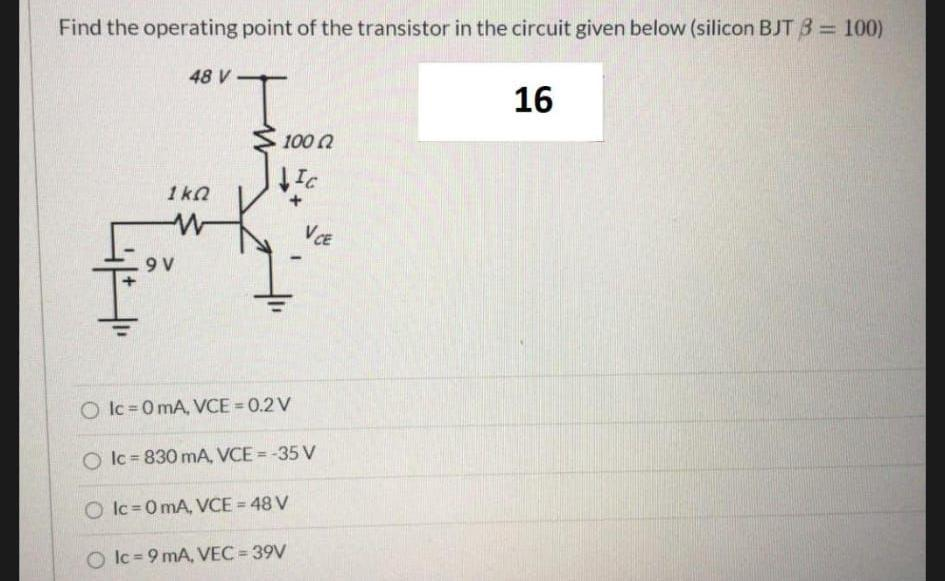Question:

# Find the operating point of the transistor in the circuit given below (silicon BJT B = 100) 48 V- 16 10002 Ic 1kn w VCE 9V O Ic=Find the operating point of the transistor in the circuit given below (silicon BJT B = 100) 48 V- 16 10002 Ic 1kn w VCE 9V O Ic=0 mA, VCE=0.2V Ic = 830 mA, VCE =-35 V O Ic=0 mA, VCE = 48 V Olc = 9 mA, VEC = 39V